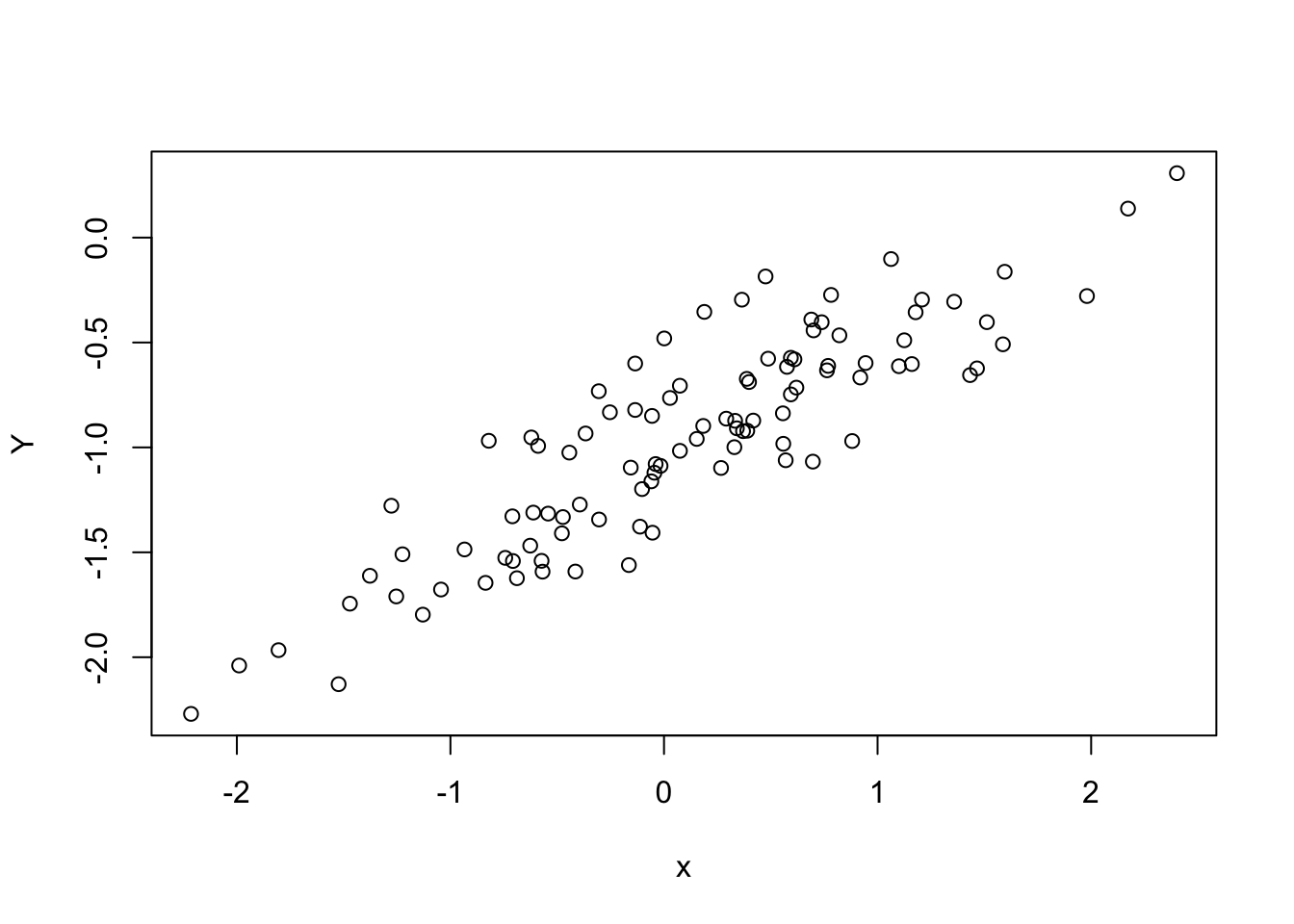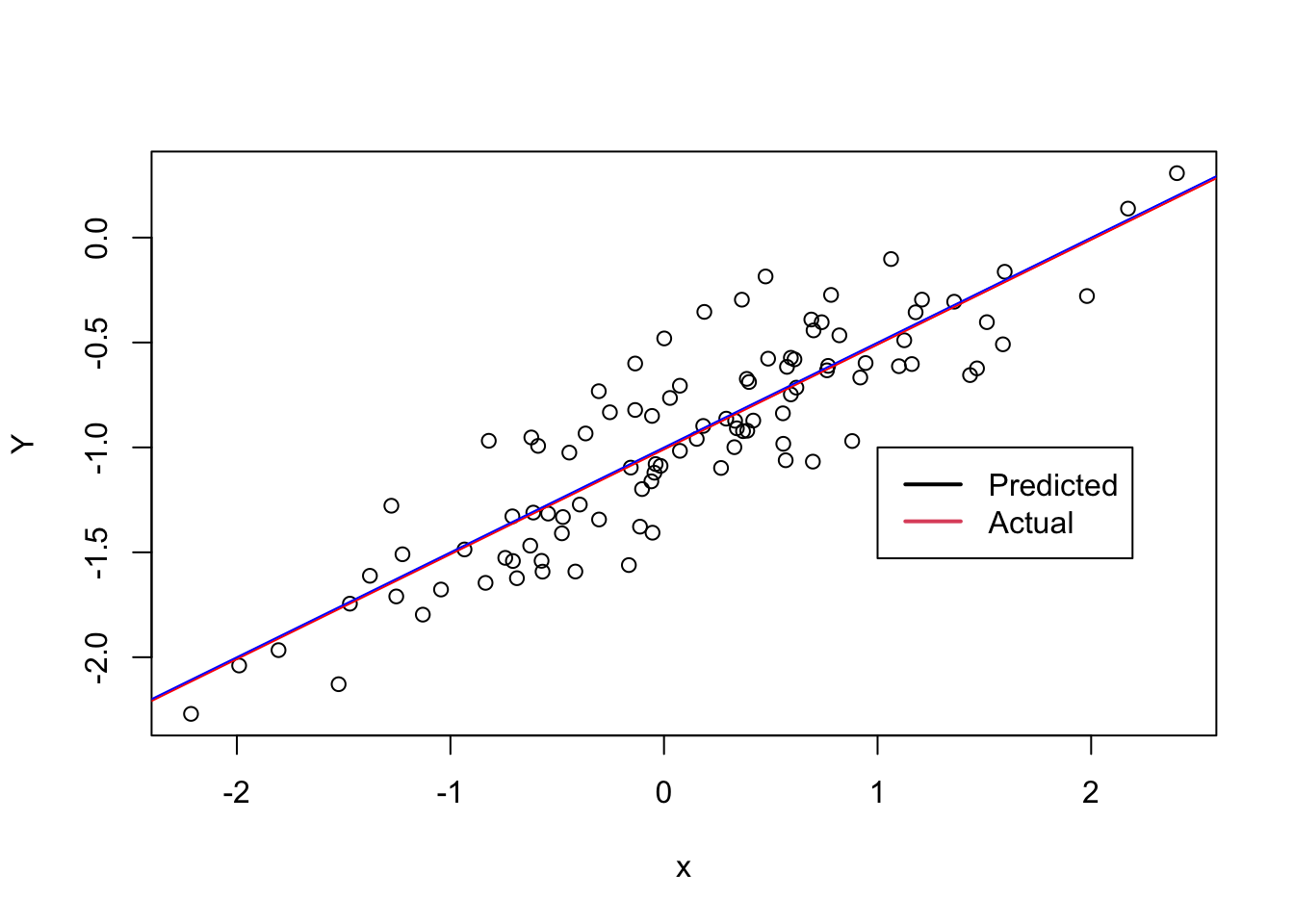ISLR Home

# Question

In this exercise you will create some simulated data and will fit simple linear regression models to it. Make sure to use set.seed(1) prior to starting part (a) to ensure consistent results

# 13a Generate 100 random normal points

Setting the seed to keep random values consistent

set.seed(1)

# Creating a vector with values drawn from a random distribution
x = rnorm(100)

# 13b Epsilon $$\epsilon \sim \mathcal{N}(0,0.25)$$

Creating a vector with values drawn from a random distribution with mean 0, standard deviation 0.25

eps = rnorm(100, 0, 0.25)

# 13c Creating the model

Y = -1 + 0.5*x + eps
• The length of vector y is 100
• $$\beta_0 = -1$$
• $$\beta_1 = 0.5$$

# 13d Plot

plot(x, Y)There is a linear relationship

# 13e

## Model Summary

lm.fit = lm(Y~x)
summary(lm.fit)
##
## Call:
## lm(formula = Y ~ x)
##
## Residuals:
##      Min       1Q   Median       3Q      Max
## -0.46921 -0.15344 -0.03487  0.13485  0.58654
##
## Coefficients:
##             Estimate Std. Error t value Pr(>|t|)
## (Intercept) -1.00942    0.02425  -41.63   <2e-16 ***
## x            0.49973    0.02693   18.56   <2e-16 ***
## ---
## Signif. codes:  0 '***' 0.001 '**' 0.01 '*' 0.05 '.' 0.1 ' ' 1
##
## Residual standard error: 0.2407 on 98 degrees of freedom
## Multiple R-squared:  0.7784, Adjusted R-squared:  0.7762
## F-statistic: 344.3 on 1 and 98 DF,  p-value: < 2.2e-16

The coefficients for estimated model are off by a few decimals

# 13f Plot

plot(x, Y)
abline(lm.fit, col='red')
abline(-1, 0.5, col="blue")
legend(-1, c("Predicted", "Actual"), col=1:2, lwd=2)# 13g Fit Model

Fitting a model introducing a quadratic term

lm.poly.fit = lm(Y~x+I(x^2))
summary(lm.poly.fit)
##
## Call:
## lm(formula = Y ~ x + I(x^2))
##
## Residuals:
##     Min      1Q  Median      3Q     Max
## -0.4913 -0.1563 -0.0322  0.1451  0.5675
##
## Coefficients:
##             Estimate Std. Error t value Pr(>|t|)
## (Intercept) -0.98582    0.02941 -33.516   <2e-16 ***
## x            0.50429    0.02700  18.680   <2e-16 ***
## I(x^2)      -0.02973    0.02119  -1.403    0.164
## ---
## Signif. codes:  0 '***' 0.001 '**' 0.01 '*' 0.05 '.' 0.1 ' ' 1
##
## Residual standard error: 0.2395 on 97 degrees of freedom
## Multiple R-squared:  0.7828, Adjusted R-squared:  0.7784
## F-statistic: 174.8 on 2 and 97 DF,  p-value: < 2.2e-16

# 13h Generating Data

Generating data randomly for x from normal distribution

x.less = rnorm(100)

Generating random error terms with small standard deviation (0.05) from normal distribution

eps.less = rnorm(100, 0, 0.05) # epsilon

# Creating the Y value given x and eps

Y.less = -1 + 0.5*x.less + eps.less

### Fit a least square regression

lm.less.fit = lm(Y.less ~ x.less)

## Model Summary

summary(lm.less.fit)
##
## Call:
## lm(formula = Y.less ~ x.less)
##
## Residuals:
##       Min        1Q    Median        3Q       Max
## -0.137090 -0.028070 -0.000874  0.033987  0.092421
##
## Coefficients:
##              Estimate Std. Error t value Pr(>|t|)
## (Intercept) -0.997578   0.004955  -201.3   <2e-16 ***
## x.less       0.505311   0.004813   105.0   <2e-16 ***
## ---
## Signif. codes:  0 '***' 0.001 '**' 0.01 '*' 0.05 '.' 0.1 ' ' 1
##
## Residual standard error: 0.04953 on 98 degrees of freedom
## Multiple R-squared:  0.9912, Adjusted R-squared:  0.9911
## F-statistic: 1.102e+04 on 1 and 98 DF,  p-value: < 2.2e-16

## Plotting the x and y values

plot(x.less, Y.less)

# Plotting the least square regression line
abline(lm.less.fit, col='red')

# Plotting the population regression line
abline(-1, 0.5, col="blue")

# Creating a legend for plot
legend(-1, c("Predicted", "Actual"), col=1:2, lwd=2)